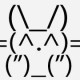# dsfsdf sdfffffffffffffffffffffffffffffffffWed Jan 10 2018 1:47:43 EST
•         Wed, 10 Jan 2018 01:47 EST

#### dsfsdf sdfffffffffffffffffffffffffffffffff

sdffffffffffffffffffffffffffffffffffff